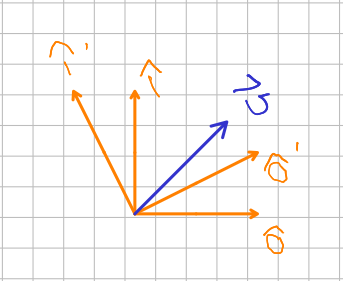# Discrete Fourier Transform¶

The discrete Fourier Transform is simply a change of coordinates. For example we have two rotated coordinate systems, the unprime system, and the prime system. We label the directions by numbers because they generalize to $N$ dimensions.We assume that the unprime coordinate system is spanned by orthogonal Cartesian vectors of N dimensions, whose elements are real numbers. The kth one will be denoted $\hat {\mathbf {k}}$ and so $\hat {\mathbf {k}} \cdot \hat {\mathbf {l}} = |\hat {\mathbf {k}}|^2\delta_{kl}$. The prime coordinates are spanned by an orthogonal basis set, denoted by $\hat {\mathbf {n'}}$, so $\hat {\mathbf {n'}} \cdot \hat {\mathbf {m'}} = |\hat {\mathbf {m'}}|^2\delta_{mn}$. The coordinates of the prime coordinate system are complex numbers. Most of us haven't done dot products with complex numbers. The property that $\mathbf{v} = |\mathbf{v}|^2$ is important and extending the dot product in a way that keeps that property and matches the convention others use is ${\mathbf {a}} \cdot {\mathbf {b}} = \sum_n a_n b_n^*$ where the star denotes complex conjugate. The vector $\mathbf b$, can be written in either prime or unprime coordinate systems. Assume we know the coordinates of $\mathbf v$ in the unprime coordinate system as well as the basis vectors, $\hat {\mathbf {k}}$ and $\hat {\mathbf {n'}}$. How do we find the prime coordinates of $\mathbf v$? $$\mathbf v = \sum_{k=0}^{N-1} v_k \hat {\mathbf {k}} = \sum_{n'=0}^{N-1} v'_{n'} \hat {\mathbf {n'}}$$ Taking the inner product on both sides with the m'th unit vector, $$\mathbf v \cdot \hat {\mathbf {m'}} = \sum_{k=0}^{N-1} v_k \hat {\mathbf {k}} \cdot \hat {\mathbf {m'}} = \sum_{n'=0}^{N-1} v'_{n'} \hat {\mathbf {n'}} \cdot \hat {\mathbf {m'}}$$ Using the orthogonality relationship for prime basis vectors, $$\mathbf v \cdot \hat {\mathbf {m'}} = \sum_{k=0}^{N-1} v_k \hat {\mathbf {k}} \cdot \hat {\mathbf {m'}} = \sum_{n'=0}^{N-1} v'_{n'} |\hat {\mathbf {m'}}|^2 \delta_{n'm'}$$ and the sifting property of the Kroniger delta yields $$\mathbf v \cdot \hat {\mathbf {m'}} = \sum_{k=0}^{N-1} v_k \hat {\mathbf {k}} \cdot \hat {\mathbf {m'}} = v'_{m'} |\hat {\mathbf {m'}}|^2$$ Dividing by $|\hat {\mathbf {m'}}|^2$ gives us what we were after. $$v'_{m'} = \frac{\mathbf v \cdot \hat {\mathbf {m'}}} {|\hat {\mathbf {m'}}|^2} = \sum_{k=0}^{N-1}\frac { v_k \hat {\mathbf {k}} \cdot \hat {\mathbf {m'}}}{|\hat {\mathbf {m'}}|^2}$$ Now let's put this to use by applying this to the situation where $$\frac{\hat {\mathbf {m'}} \cdot \hat {\mathbf {k}}}{|\hat {\mathbf {m'}}|^2} = e^{j2 \pi n'k/N}$$ This is saying that the components of the prime vector, $\hat {\mathbf {m'}}$ along the unprime kth direction are proportional to $e^{j2\pi n'k/N}$. I leave it as an execrise for the reader to determine the constant of proportionality. If you want to tackle this, a useful hint is: $\hat {\mathbf {n'}} \cdot \hat {\mathbf {m'}} = \delta_{mn} |\hat {\mathbf {m'}}|^2$. (Hint: use $x = e^{j2 \pi k/N}$ and the geometric sum $\sum_{n'=0}^{N-1} x^{n'} = \frac{1-x^N}{1-x}$ when $n' \neq m'$.) From all this, you get $$v'_{m'} = \frac{\mathbf v \cdot \hat {\mathbf {m'}}} {|\hat {\mathbf {m'}}|^2} = \sum_{k=0}^{N-1}\frac { v_k \hat {\mathbf {k}} \cdot \hat {\mathbf {m'}}}{|\hat {\mathbf {m'}}|^2} = \sum_{k=0}^{N-1}\ v_k e^{-j2 \pi m'k/N}$$ The negative sign on the complex exponential comes from the complex conjugate operation for the second vector.

## The Discrete Fourier Transform¶

The kth element of a sequence of data taken by an A/D converter reading a signal, $h(t)$ at intervals $T$ seconds apart at times, $t \in \{0, T, 2T, 3T, ..., (N-1)T\}$ is $h(kT)$, known more simply as $h(k)$. The Discrete Fourier Transform (DFT) is a change of basis set similar to the one shown above where $m' = n$, $v'_{m'} = H(n)$ and $v_k = h(k)$. The DFT of $h(k)$ is known as $H(n)$ and has the same number of dimensions as the sampled time signal, $N$. $$n \in \{0, 1, 2, 3, ..., N-1\}$$ $$H(n) = \sum_{k=0}^{N-1} h(k) e^{-j2\pi nk/N}$$ The reverse transformation from $H(n)$ to $h(k)$ is given by the Inverse Discrete Fourier Transform: $$h(k) = 1/N\sum_{n=0}^{N-1} H(n) e^{j2\pi nk/N}$$ The DFT is not usually derived this way because it is actually an approximation to the continuous Fourier Transform as we shall see later this quarter. However, this should show you that it is a also a simple change of basis set to go from $h(k)$ to $H(n)$. The same vector $h(k)$ is just described in a different way. The notation used above, especially that for the components of the vectors will be very useful, because it allows us to easily work with $N$ dimensional vectors.

Here are some example DFTs using python.

In :
import numpy as np
import matplotlib.pyplot as plt

h1 = [1, 0, -1, 0]
print(h1)
print('H = ' + str(np.fft.fft(h1)))
h2 = [0, 1, 0, -1]
print(h2)
print('H = ' + str(np.fft.fft(h2)))
h3 = [1, 1, 1, 1]
print(h3)
print('H = ' + str(np.fft.fft(h3)))

[1, 0, -1, 0]
H = [0.+0.j 2.+0.j 0.+0.j 2.+0.j]
[0, 1, 0, -1]
H = [0.+0.j 0.-2.j 0.+0.j 0.+2.j]
[1, 1, 1, 1]
H = [4.+0.j 0.+0.j 0.+0.j 0.+0.j]


## Symmetries of the DFT¶

• The DFT is periodic with period N. $$H(n+N) = \sum_{k=0}^{N-1} h(k) e^{-j2\pi (n+N)k/N} = \sum_{k=0}^{N-1} h(k) e^{-j2\pi nk/N} e^{j2\pi k} = \sum_{k=0}^{N-1} h(k) e^{-j2\pi nk/N} = H(n)$$ because $e^{j2\pi k} = 1$.
• The IDFT is also periodic with period N. $$h(k+N) = 1/N\sum_{n=0}^{N-1}e^{-j2\pi n(k+N)/N} = 1/N\sum_{n=0}^{N-1}e^{-j2\pi nk/N} e^{j2\pi n} = h(k)$$
• If $h(k) \in \mathscr R$, coefficients for negative $n$ (frequencies) are complex conjugates of those for positive $n$. $$H(-n) = \sum_{k=0}^{N-1} h(k) e^{j2\pi nk/N} = \left [ \sum_{k=0}^{N-1} h(k) e^{-j2\pi nk/N} \right]^* = H(n)^*$$
• The DFT of a real even function of $k$ is real, and the DFT of a real odd function of $k$ is imaginary. Note that h1 h2, and h3 above follow this pattern. I leave the proof of this as an exercise for the reader. You have to use Euler, and a clever change of limits on the sum so it is symmetric around zero.
In [ ]: